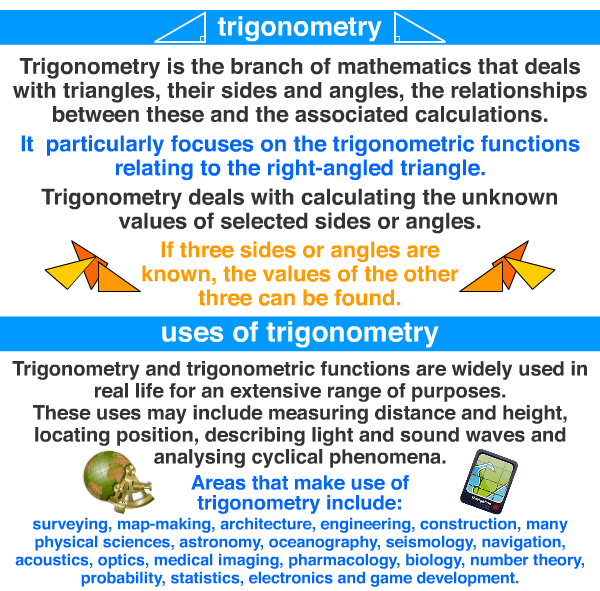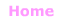Aa Bb Cc Dd Ee Ff Gg Hh Ii Jj Kk Ll Mm Nn Oo Pp Qq Rr Ss Tt Uu Vv Ww Xx Yy Zz

Tt

trigonometry

• the branch of mathematics that deals with triangles
and their sides and angles.
• the study of the relationships between
the sides and angles of triangles, and
the associated calculations,
particularly trigonometric functions
relating to right-angled triangles.

EXAMPLE:more

Basic Trigonometry ~ Video

Graphs of Trigonometric Functions
http://www.intmath.com/trigonometric-graphs/trigo-graph-intro.php

Trigonometry
https://en.wikipedia.org/wiki/Trigonometry

Trigonometric Functions
https://en.wikipedia.org/wiki/Trigonometric_functions© Jenny Eather 2014. All rights reserved.